## Brayton Cycle – Process, PV Diagram and TS Diagram:

The Brayton cycle represents the operation of a gas turbine engine. The cycle consists of four processes. This cycle was invented by an American Engineer George Baily Brayton. Brayton cycle describes the working of a constant pressure heat engine. Gas turbine and air breathing jet engine use the Brayton cycle. Although the Brayton cycle is usually run as a open cycle system, it is conventual assumed for the purpose of thermodynamic analysis that the exhaust gases are reused in the intake, therefore enabling analysis as a closed system.

The main components of a gas turbine are:

1. Compressor
2. Combustion chamber
3. Turbine
4. Condenser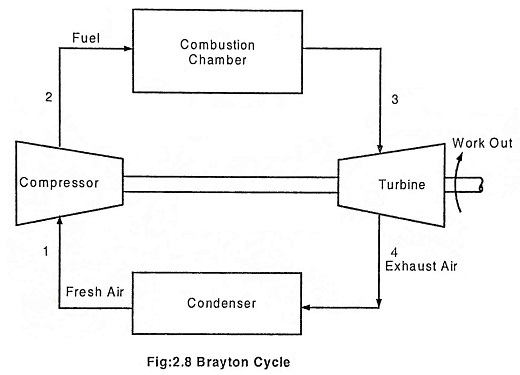### Process 1 – 2 Isentropic compression

The ambient air is drawn to the compressor and is pressurized or compressed from pressure P1 to P2. The temperature increases from T1 to T2, volume reduces from V1 to V2 with the entropy being constant.

### Process 2 – 3 Heat addition at constant pressure

The compressed air then runs through a combustion chamber, where fuel is burned, heat is added at constant pressure P2 = P3. Temperature rises from T2 to T3 Due to the combustion, volume increases from V2 to V3 and the entropy also increases from S2 to S3.

### Process 3 – 4 Isentropic expansion

The heated, pressurized air then gives up its energy by expanding through a turbine. The work extracted by the turbine is used to drive the compressor which is coupled to it. The pressure decreases from P3 to P4. Temperature decreases from T3 to T4. Volume slightly increases from V3 to V4, and the entropy remains constant S3 = S4.

### Process 4 – 1 Heat rejection at constant pressure

In an open Brayton cycle the exhaust air is released to the atmosphere and fresh air is drawn by the compressor for the next cycle, where as in closed Brayton cycle, the exhaust air is condensed in a condenser and then redrawn by the compressor. Heat is rejected at constant pressure P4 = P1 in both the cases. The temperature decreases from T4 to T1, volume decreases from V4 to V1 and the entropy decreases from S4 to S1.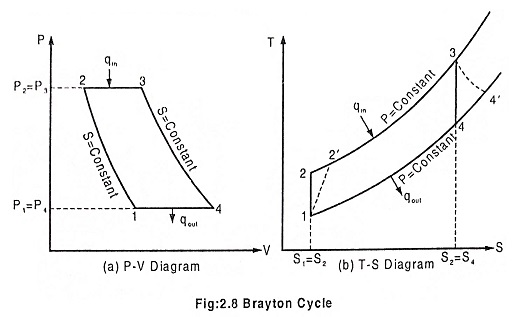Thermal efficiency of the cycle

Heat supplied at constant pressure = m Cp (T3 – T2)

Heat rejected at constant pressure = m Cp (T4 – T1)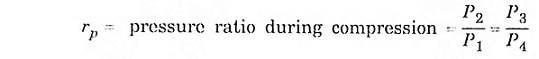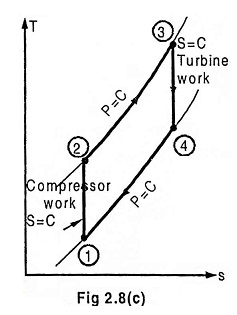Process (1) – (2) isentropic process  (3) – (4) isentropic process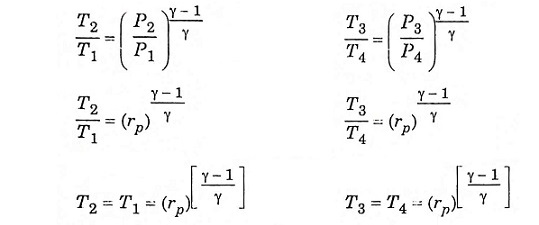Substitute T2 and T3 in eqn (1), we get ηAir standard (or) ηthermal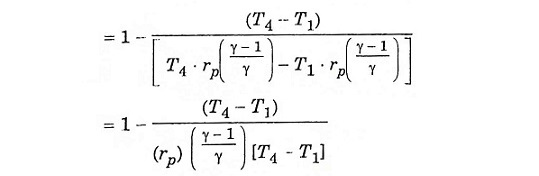Scroll to Top